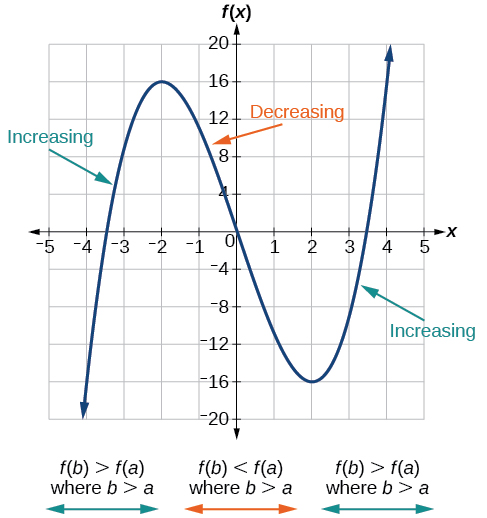3.3 Rates of change and behavior of graphs  (Page 3/15)

 Page 3 / 15

Find the average rate of change of $\text{\hspace{0.17em}}f\left(x\right)={x}^{2}+2x-8\text{\hspace{0.17em}}$ on the interval $\text{\hspace{0.17em}}\left[5,a\right]\text{\hspace{0.17em}}$ in simplest forms in terms
of $\text{\hspace{0.17em}}a.$

$\text{\hspace{0.17em}}a+7\text{\hspace{0.17em}}$

Using a graph to determine where a function is increasing, decreasing, or constant

As part of exploring how functions change, we can identify intervals over which the function is changing in specific ways. We say that a function is increasing on an interval if the function values increase as the input values increase within that interval. Similarly, a function is decreasing on an interval if the function values decrease as the input values increase over that interval. The average rate of change of an increasing function is positive, and the average rate of change of a decreasing function is negative. [link] shows examples of increasing and decreasing intervals on a function.The function   f ( x ) = x 3 − 12 x   is increasing on   ( − ∞ ,   − 2 ) ∪ ​ ​ ( 2 ,   ∞ )   and is decreasing on   ( − 2 ,   2 ) .

While some functions are increasing (or decreasing) over their entire domain, many others are not. A value of the input where a function changes from increasing to decreasing (as we go from left to right, that is, as the input variable increases) is called a local maximum    . If a function has more than one, we say it has local maxima. Similarly, a value of the input where a function changes from decreasing to increasing as the input variable increases is called a local minimum    . The plural form is “local minima.” Together, local maxima and minima are called local extrema    , or local extreme values, of the function. (The singular form is “extremum.”) Often, the term local is replaced by the term relative . In this text, we will use the term local .

Clearly, a function is neither increasing nor decreasing on an interval where it is constant. A function is also neither increasing nor decreasing at extrema. Note that we have to speak of local extrema, because any given local extremum as defined here is not necessarily the highest maximum or lowest minimum in the function’s entire domain.

For the function whose graph is shown in [link] , the local maximum is 16, and it occurs at $\text{\hspace{0.17em}}x=-2.\text{\hspace{0.17em}}$ The local minimum is $\text{\hspace{0.17em}}-16\text{\hspace{0.17em}}$ and it occurs at $\text{\hspace{0.17em}}x=2.$

To locate the local maxima and minima from a graph, we need to observe the graph to determine where the graph attains its highest and lowest points, respectively, within an open interval. Like the summit of a roller coaster, the graph of a function is higher at a local maximum than at nearby points on both sides. The graph will also be lower at a local minimum than at neighboring points. [link] illustrates these ideas for a local maximum.

These observations lead us to a formal definition of local extrema.

Local minima and local maxima

A function $\text{\hspace{0.17em}}f\text{\hspace{0.17em}}$ is an increasing function    on an open interval if $\text{\hspace{0.17em}}f\left(b\right)>f\left(a\right)\text{\hspace{0.17em}}$ for any two input values $\text{\hspace{0.17em}}a\text{\hspace{0.17em}}$ and $\text{\hspace{0.17em}}b\text{\hspace{0.17em}}$ in the given interval where $\text{\hspace{0.17em}}b>a.$

A function $\text{\hspace{0.17em}}f\text{\hspace{0.17em}}$ is a decreasing function    on an open interval if $\text{\hspace{0.17em}}f\left(b\right) for any two input values $\text{\hspace{0.17em}}a\text{\hspace{0.17em}}$ and $\text{\hspace{0.17em}}b\text{\hspace{0.17em}}$ in the given interval where $\text{\hspace{0.17em}}b>a.$

A function $f$ has a local maximum at $\text{\hspace{0.17em}}x=b$ if there exists an interval $\text{\hspace{0.17em}}\left(a,c\right)$ with $a such that, for any $x$ in the interval $\left(a,c\right),$ $f\left(x\right)\le f\left(b\right).$ Likewise, $f$ has a local minimum at $x=b$ if there exists an interval $\left(a,c\right)$ with $a such that, for any $x$ in the interval $\left(a,c\right),$ $f\left(x\right)\ge f\left(b\right).$

sin^4+sin^2=1, prove that tan^2-tan^4+1=0
what is the formula used for this question? "Jamal wants to save \$54,000 for a down payment on a home. How much will he need to invest in an account with 8.2% APR, compounding daily, in order to reach his goal in 5 years?"
i don't need help solving it I just need a memory jogger please.
Kuz
A = P(1 + r/n) ^rt
Dale
how to solve an expression when equal to zero
its a very simple
Kavita
gave your expression then i solve
Kavita
Hy guys, I have a problem when it comes on solving equations and expressions, can you help me 😭😭
Thuli
Tomorrow its an revision on factorising and Simplifying...
Thuli
ok sent the quiz
kurash
send
Kavita
Hi
Masum
What is the value of log-1
Masum
the value of log1=0
Kavita
Log(-1)
Masum
What is the value of i^i
Masum
log -1 is 1.36
kurash
No
Masum
no I m right
Kavita
No sister.
Masum
no I m right
Kavita
tan20°×tan30°×tan45°×tan50°×tan60°×tan70°
jaldi batao
Joju
Find the value of x between 0degree and 360 degree which satisfy the equation 3sinx =tanx
what is sine?
what is the standard form of 1
1×10^0
Akugry
Evalute exponential functions
30
Shani
The sides of a triangle are three consecutive natural number numbers and it's largest angle is twice the smallest one. determine the sides of a triangle
Will be with you shortly
Inkoom
3, 4, 5 principle from geo? sounds like a 90 and 2 45's to me that my answer
Neese
answer is 2, 3, 4
Gaurav
prove that [a+b, b+c, c+a]= 2[a b c]
can't prove
Akugry
i can prove [a+b+b+c+c+a]=2[a+b+c]
this is simple
Akugry
hi
Stormzy
x exposant 4 + 4 x exposant 3 + 8 exposant 2 + 4 x + 1 = 0
x exposent4+4x exposent3+8x exposent2+4x+1=0
HERVE
How can I solve for a domain and a codomains in a given function?
ranges
EDWIN
Thank you I mean range sir.
Oliver
proof for set theory
don't you know?
Inkoom
find to nearest one decimal place of centimeter the length of an arc of circle of radius length 12.5cm and subtending of centeral angle 1.6rad
factoring polynomialByByBy